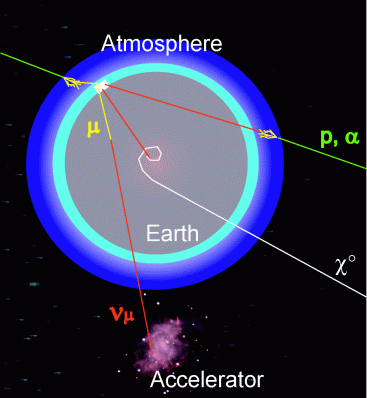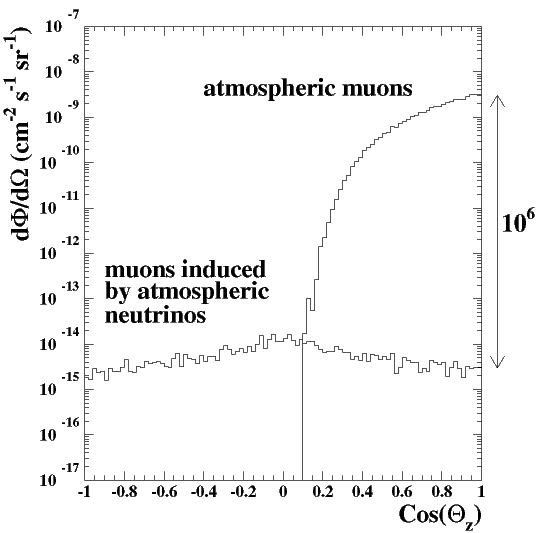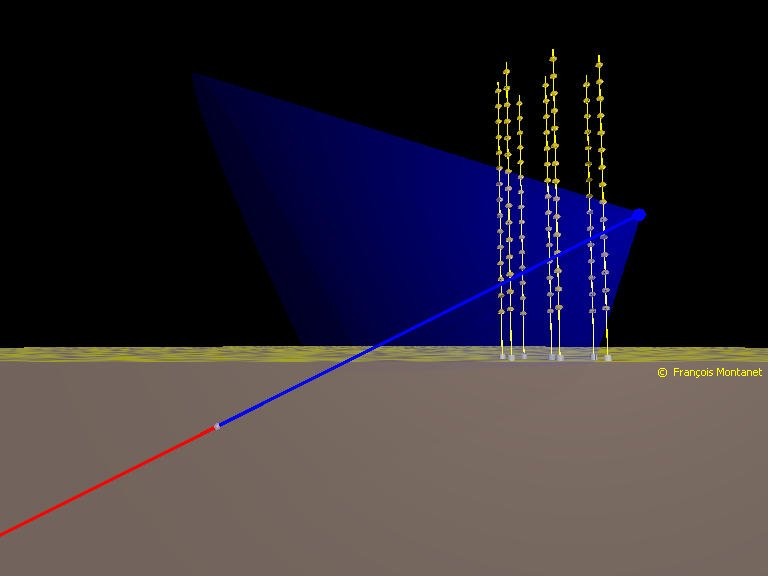# Detection principle

The main ingredients necessary for the detection of a neutrino in a neutrino telescope are nicely illustrated in this video : 1) the transparency of Earth to neutrinos 2) a neutrino interaction in the vicinity of the detector 3) Cherenkov light emission in sea water

(credit: Alexander Kappes)

Since the Earth acts as a shield against all particles except neutrinos, a neutrino telescope uses the detection of upward-going charged particles as a signature of neutrino interactions in the matter below or inside the detector (figure left). This detection technique requires discriminating upward going particles against the much higher flux of downward atmospheric muons (figure right).In order to correlate the measured muon spectrum with the original neutrino spectrum, it is necessary to understand the dynamics of neutrino interactions, the opacity of the Earth, the energy loss of muons and the resolution of the detector over a wide range of angles and energies.

## Different types of neutrino interactions in ANTARES

• Charged-current $$\nu_e$$ interactions give rise to electromagnetic and hadronic showers with longitudinal dimensions of no more than a few metres, because the radiation length and the nuclear interaction length of water are below 1 m. On the scale of ANTARES, these are nearly point-like events. At energies above 100 GeV, the energy resolution of these events is expected to be better than for muon events because they leave all of their energy inside the detector volume. On the other hand, their angular resolution will be poor compared to muon events, due to the point-like character of the showers. Charged-current $$\nu_e$$ interactions will be contaminated by neutral-current interactions of both $$\nu_e$$ and $$\nu_\mu$$ (and $$\nu_\tau$$, if present).
The number of neutral-current interactions is about 1/3 of the number of charged-current interactions. The neutrino type is not identified in the neutral-current interactions, the energy resolution is poor due to the missing final-state neutrino, and the angular resolution is poor due to the point-like character.

• Charged-current $$\nu_\mu$$ interactions produce $$\mu^\pm$$ leptons as well as a point-like hadronic shower. The $$\nu_\mu$$ energy can be estimated from the measured $$\mu^\pm$$ energy. The $$\mu^\pm$$ energy can be determined from the range for E<100 GeV, or from dE/dx for E>1TeV. For $$\nu_\mu$$ interactions inside the detector, additional information on the $$\nu_\mu$$ energy is available from the hadronic shower.

• Charged-current $$\nu_\tau$$ interactions produce $$\tau^\pm$$ leptons with electronic, muonic and hadronic decay modes. The $$\nu_\tau$$ interaction vertex and the $$\tau^\pm$$ decay vertex cannot be separated for energies below around 100 TeV. The electronic and hadronic modes will look like $$\nu_e$$ charged-current or neutral-current interactions. The muonic decays, with branching ratio 17%, cannot be distinguished from $$\nu_\mu$$ charged-current interactions.

## Cherenkov light emission

Charged particles emit light under a characteristic angle when passing through a medium if their velocity exceeds the speed of light in the medium.In the energy range interesting for ANTARES (E > 10 GeV), particles will generally be ultra-relativistic therefore the Cherenkov light is emitted under $$42^\circ$$ for blue light. This easy geometrical pattern of light emission allows a precise reconstruction of tracks from the measurement of only few hits at different space points.

At wavelengths of 400-500 nm the efficiency of the photomultipliers as well as the transparency of the water are maximal. Within 1 cm flight path 100 photons are emitted in this wavelength bin. Between 285-400 nm twice as many photons are emitted, however they contribute less to the detected signal. At a perpendicular distance of 40 m from a charged track the density of photons between 400-500 nm is still 1 per 340 cm2, neglecting absorption and scattering effects. The effective area of the used photomultipliers is in the same range (300-500 cm2). This gives an indication of the active detector volume around each photomultiplier.

For ultra-relativistic particles the Cherenkov light yield is independent of the energy of the charged particle. This means the light output of a single particle does not allow its energy to be measured. However when hadronic or electromagnetic showers are produced (which might occur at the neutrino vertex as well as for radiative processes along a muon track) the total light yield of the shower will be proportional to the total track length in the shower and therefore to its initial energy. This allows some calorimetric measurements if the neutrino vertex is inside the active detector volume or for muon tracks above 1 TeV where radiative processes dominate its energy loss.

## Observable sky

The ANTARES neutrino telescope, situated at a latitude of 43° North, can observe upward-going neutrinos from most of the sky (about $$3.5\pi$$ sr), due to the rotation of the Earth. Declinations below -47° are always visible, while those above +47° are never visible. Declinations between -47° and +47° are visible for part of the sidereal day. Most of the Galactic plane is visible, and the Galactic centre is visible most of the sidereal day.

At energies greater than about 40 TeV, the interaction length becomes smaller than the Earth’s diameter for neutrinos traversing the dense core of the Earth. Above 10 PeV, only nearly horizontal neutrinos are visible. If the field of view can be extended to 10° above the horizon at these energies where the background is greatly diminished, a non-negligible fraction of the sky can be kept observable even at these energies.